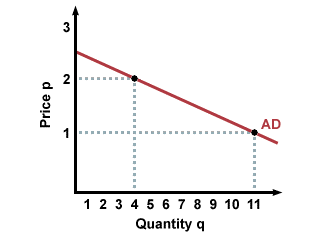A summation strategy that breaks down the summands by taking note of the positional value and continuing with the addition

## What is Horizontal Addition?

The horizontal addition is a summation strategy that breaks down the summands by taking note of the positional value and continuing with the addition. The sums in the addition chain do not mix the positional values, making it easier for students to comprehend the addition. When adding the broken-down values, the thousands are added to the thousands, the hundreds are added to the hundreds, and the tens to the tens, etc.

### How Horizontal Addition Works (Example)

When doing a horizontal addition, the first step is to decompose the summands and then continue with the addition chain. Let’s use the following addition example:

4553 + 1424 = ?

The first step when calculating the summation is to use the composition and addition chain method as follows:

Step 1: Break down the summands

4553 = 4000 + 500 + 50 + 3

1424 = 1000 + 400 + 20 + 4

Step 2: Add the decomposed numbers

= (4000 + 1000) + (500 + 400) + (50 + 20) + (3 + 4)

= 5000 + 900 + 70 + 7

= 5977

Alternatively,

You can break down only one of the summands, and add the broken-down numbers to the other summand as shown below:

4553 + 1424 = ?

4553 = 4000 + 500 + 50 + 3

Total = 1424 + 4000 + 500 + 50 +3

= 5977

### The Market Demand Curve for Private and Public Goods

The concept of horizontal addition also comes into effect when determining the market demand curve for a public and private good. Public goods are defined as non-excludable goods that an individual cannot be excluded from receiving their benefits. Also, when an individual utilizes the public good, it does not affect the good’s availability to other consumers.

An example of a public good is a public park and street lights. On the other hand, a private good is a good that is limited in quantity, and its holders/owners can limit other individuals from enjoying its benefits. Due to its limited availability, the individual must make a payment in order to enjoy its benefits. An example of a private good is food, water, houses, etc.

#### 1. Private Goods (Horizontal Addition)

Private goods are limited in supply, and their market demand curve is obtained by horizontally summing the individual demand curves. Unlike public goods, private goods are rivals in consumption, which means that their demand is calculated by adding the quantity that potential consumers are willing to pay at a specific price. The focus of the private goods market demand is the actual price that a customer is willing to pay for a specific quantity of goods.

For example, if the market for French fries has two buyers, Peter and John, we would add the prices that they are willing to buy at price P1 for a specific quantity of the good. This would then be recorded as the aggregate demand for P1. Then, we would add the specific quantity that the two buyers are willing to buy at price P2 and record it as aggregate demand for P2. The aggregate demand curve for French fries would be as follows:The market demand for private goods is obtained through horizontal addition because you need to look at the price level and the quantities of goods demanded at each level and obtain the total quantity that the two buyers demanded at the price level.

#### 2. Public Goods (Vertical Addition)

A public good is non-excludable, and it is obtained by vertically summing the individual demand curves. Since public goods are non-rivals in consumption, the value of the goods can be calculated by adding together the value that individuals obtain from consuming a specific quantity of the good. The non-rival character of public goods makes their deviation from the actual demand vary from that of private goods.

To illustrate the market demand for public goods, let’s take an example of two individuals, Peter and John, who are enjoying the benefits of a public park, a public good. The two individuals are of different economic capabilities and are willing and able to purchase the public good.

Assume that Peter is willing and able to pay \$10 when enjoying the park, while John is willing to pay up to \$20 for the same good. The total market demand equals the amount that the two individuals are willing and able to pay for using the park, which is \$30 (\$10 + \$20) in this case. The market demand curve is derived by summing the prices that both Peter and John are willing to pay for consuming specific quantities of the public good. The prices are vertically added for a given quantity of the good.

### More Resources

CFI is the official provider of the global Financial Modeling & Valuation Analyst (FMVA)™ certification program, designed to help anyone become a world-class financial analyst. To keep advancing your career, the additional resources below will be useful:

• Aggregate Supply and Demand